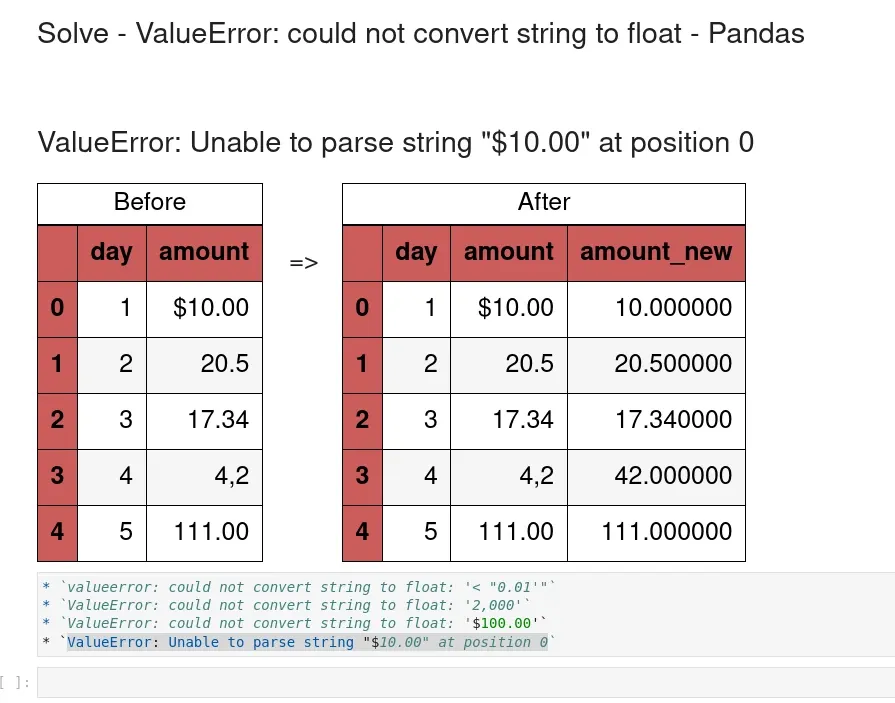In this short guide, I'll show you how to solve Pandas or Python errors:

• `valueerror: could not convert string to float: '< "0.01'"`
• `ValueError: could not convert string to float: '2,000'`
• `ValueError: could not convert string to float: '\$100.00'`
• `ValueError: Unable to parse string "\$10.00" at position 0`

We will see how to solve the errors above and how to identify the problematic rows in Pandas.

## Setup

Let's create an example DataFrame in order to reproduce the error:

ValueError: could not convert string to float: '\$10.00'

``````import pandas as pd

df = pd.DataFrame({'day': [1, 2, 3, 4, 5],
'amount': ['\$10.00', '20.5', '17.34', '4,2', '111.00']})
``````

DataFrame looks like:

day amount
0 1 \$10.00
1 2 20.5
2 3 17.34
3 4 4,2
4 5 111.00

## Step 1: ValueError: could not convert string to float

To convert string to float we can use the function: `.astype(float)`. If we try to do so for the column - amount:

``````df['amount'].astype(float)
``````

we will face error:

``````ValueError: could not convert string to float: '\$10.00'
``````

## Step 2: ValueError: Unable to parse string "\$10.00" at position 0

We will see similar result if we try to convert the column to numerical by method `pd.to_numeric(`:

``````pd.to_numeric(df['amount'])
``````

error is raised:

``````ValueError: Unable to parse string "\$10.00" at position 0
``````

In both cases we can see the problematic value. But we are not sure if more different cases exist.

## Step 3: Identify problematic non numeric values

To find which values are causing the problem we will use a simple trick. We will convert all values except the problematic ones by:

``````pd.to_numeric(df['amount'], errors='coerce')
``````

This give us successfully converted float values:

``````0       NaN
1     20.50
2     17.34
3       NaN
4    111.00
Name: amount, dtype: float64
``````

The ones in error will be replaced by `NaN`. No we can use mask to get only value which cause the error during the conversion to numerical values:

``````df[pd.to_numeric(df['amount'], errors='coerce').isna()]['amount']
``````

result is:

``````0    \$10.00
3       4,2
Name: amount, dtype: object
``````## Step 4: Solve ValueError: could not convert string to float

To solve the errors:

ValueError: could not convert string to float: '\$10.00'
ValueError: Unable to parse string "\$10.00" at position 0

We have several options:

• ignore errors from invalid parsing and keep the output as it is:
• `pd.to_numeric(df['amount'], errors='ignore')`
• convert only numeric values and `NaN` for the rest in the column:
• `pd.to_numeric(df['amount'], errors='coerce')`
• fix problematic values

In this step we are going to fix the problematic values. This can be done by replacing the non numeric symbols like:

• `\$`
• `,` - wrong decimal symbol etc

So to replace the problematic characters we can use `str.replace`:

``````df['amount'].str.replace('\$', '', regex=True)
``````

Replacing multiple characters can be done by chaining multiple functions like.(for multiple replacing values):

``````df['amount'].str.replace('\$', '', regex=True).replace('\,', '.', regex=True)
``````

of by using regex (when all symbols are replaced by a single value):

``````df['amount'].str.replace('[\$|,]', '', regex=True)
``````

Finally we get only numeric values which can be converted to numeric column:

0 10.00
1 20.5
2 17.34
3 42
4 111.00
Name: amount, dtype: object

## Step 5: Convert numbers and keep the rest

Finally if we like to convert only valid numbers we can use `errors='coerce'`. Then for all missing values we can populate them from the original column or Series:

``````data = pd.Series(['\$10.00', '20.5', '17.34', '4,2', '111.00', np.NaN, ''])
conv_data = pd.to_numeric(data, errors='coerce').fillna(data)
conv_data
``````

the result will be:

``````0    \$10.00
1      20.5
2     17.34
3       4,2
4     111.0
5       NaN
6
dtype: object
``````

While using

``````pd.to_numeric(['\$10.00', '20.5', '17.34', '4,2', '111.00', np.NaN, ''], errors='ignore')
``````

will keep all the values the same if there is invalid parsing:

``````array(['\$10.00', '20.5', '17.34', '4,2', '111.00', nan, ''], dtype=object)
``````

## Conclusion

In this post, we saw how to properly convert strings to float columns in Pandas. We covered the most popular errors and how to solve them.

Finally we discussed finding the problematic cases and fixing them.